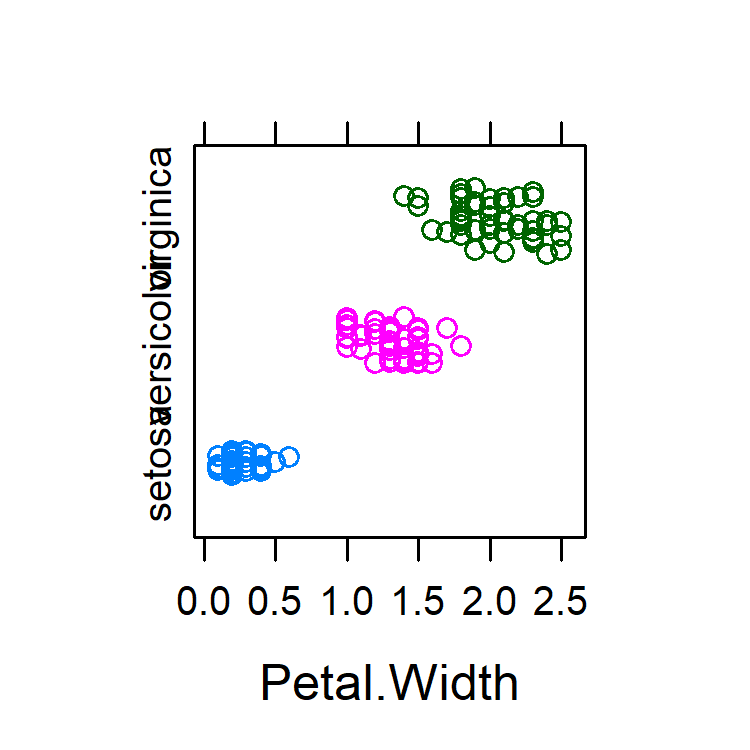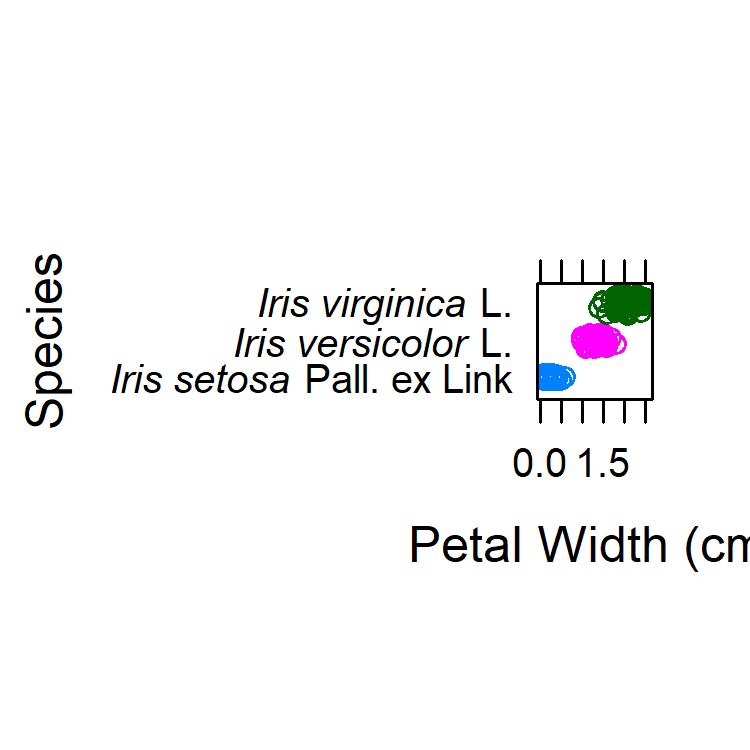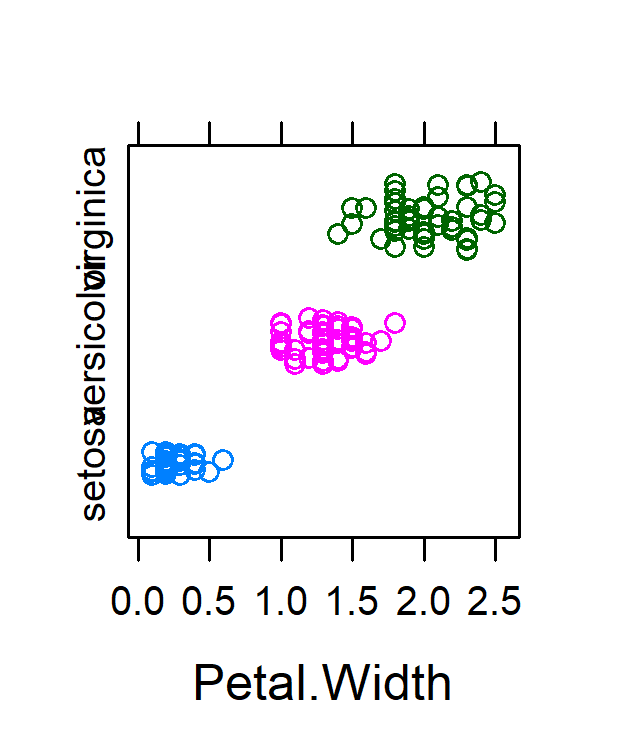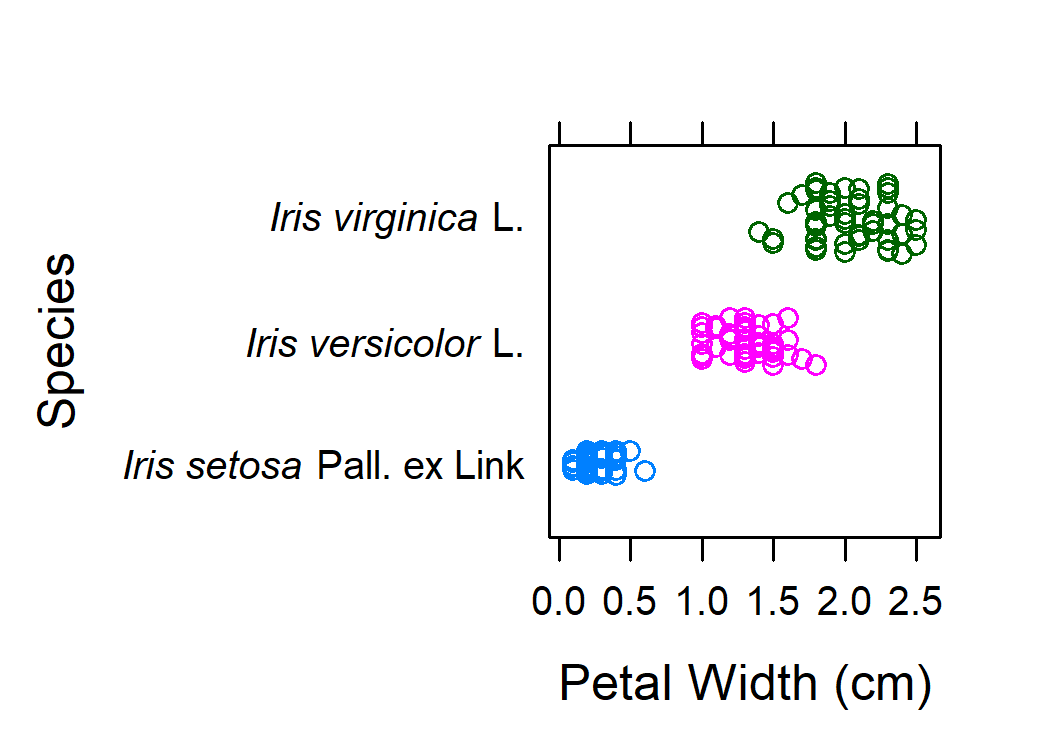Scale A Graphics Device by Plot Dimensions

Summary

When R generates figures, marginal text is normally plotted full-scale on a device with pre-determined size, and the plot area is reduced as necessary. The plotscale package reverses the dependency, so that the plot area is pre-determined and the device size is inflated as necessary.

Example

Let’s plot iris data twice, with and without really formal species labels, using small square devices.

library(plotscale)
library(lattice)
data("iris")

file1 <- paste0(tempfile(),'.png')
file2 <- paste0(tempfile(),'.png')

fig1 <- stripplot(
Species~Petal.Width, data = iris,
groups = Species, #gratuitous color
scales = list(y=list(rot = 90)),
jitter.data = TRUE, factor = 5, aspect = 1)

fig2 <- stripplot(
paste('Iris', Species)~Petal.Width, data = iris,
groups = Species,
xlab = 'Petal Width (cm)', ylab = 'Species',
jitter.data = TRUE, factor = 5, aspect = 1,
scales = list(y = list(labels = c(
expression(italic('Iris versicolor')*' L.'),
expression(italic('Iris virginica')*' L.')))))

as.png(fig1, filename = file1, width = 2.5, height = 2.5, scaled = FALSE)
as.png(fig2, filename = file2, width = 2.5, height = 2.5, scaled = FALSE)In the first figure (contrived), y labels are overlapping. In the second figure, labels are rotated, but now the plot area (coordinate plane) is squashed by marginal material. Notice also that a square device (aspect ratio 1) is wider than necessary for the first figure and taller than necessary for the second. Manual experimentation with other values of width and height would be helpful but tedious.

Solution

Instead of specifying device dimensions directly, we can let them be a function of desired plot size. Checking our original figure, we can calculate the effective dimensions of the plot area for the original device.

(ps <- plotsize(fig1, width = 2.5, height = 2.5))
## width 1.308 in , height 1.308 in

We can also calculate what device size would be needed to give these plot dimensions.

devsize(fig1, width = ps$width, height = ps$height)
## width 2.06 in , height 2.5 in

Now we re-plot the figures. By default (scaled = TRUE), width and height are understood as plot dimensions, and device dimensions are scaled accordingly.

width <- ps$width height <- ps$height
file3 <- paste0(tempfile(),'.png')
file4 <- paste0(tempfile(),'.png')
as.png(fig1, filename = file3, width = width, height = height)
as.png(fig2, filename = file4, width = width, height = height)For these two figures, the dimensions of the coordinate plane are the same. as.png() has created a device with whatever dimensions necessary to achieve the intended plot size. as.pdf() (not shown) works the same way to create PDF output. This means we can add labels, legends, and marginal text without compromising the proportions of plot elements. Furthermore, there is never wasted space in either device dimension for fixed-aspect figures.

Discussion

Device-centric figure sizing makes sense for media with real physical constraints, such as paper. Physical constraints are less an issue for virtual documents. In many contexts, actual physical dimensions of a figure matter very little since the figure is rescaled anyway to fit available screen or page space. For dynamically-rescaled figures, plot-centric sizing makes sense: it gives the user consistent control over the relative sizes of plot elements.

Details

In theory it should be possible to calculate deterministically the relationships among plot space and marginal material. plotscale use an empirical approach, iteratively creating test devices to optimize the dimensions of the first panel (i.e, the first viewport in the rendered display with ‘panel’ in its name). For more details on optimization, try devsize(..., verbose = T).

It is relatively straightforward to find solutions for figures with independent width and height; fixed-aspect figures are more problematic. For such figures, requested width and height are automatically adjusted as necessary to maintain aspect, and the solution ensures that there is no superfluous marginal space in either dimension.